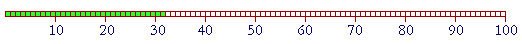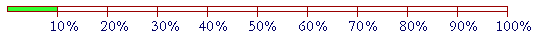S k i l l
i n
A R I T H M E T I C

Lesson 4  Section 2

# The Meaning of Percent

The student should first understand Section 1: Multiplying and Dividing by Powers of 10.

 5. What does "percent" mean? Percent is an abbreviation for the Latin per centum, which means for each 100.

100% , then, means 100 for each 100, which is all.  100% of 12 is 12.

50% is another way of saying half because 50% means 50 for each 100, which is half.  50% of 12 is 6.

A percent means how many for each 100.

Example 1.   Below are 100 small squares, and 32 have been shaded.What percent of the squares have been shaded?

Answer.   32% -- 32 for each 100.

If we think of a quantity being divided into one hundred equal parts, that is, into hundredths, then a percent is a number of hundredths. 32% is 32 hundredths.

When the percent is less than or equal to 100%, then we can say "out of" 100.  32% is 32 out of 100.  But to say that 200% is 200 out of 100 makes no sense.  200% is 200 for each 100, which is to say, twice as much.

200% of 12 is 24.

Example 2.   100 people were surveyed, and 65 responded Yes.  What percent responded Yes?

Answer.   65% -- 65 out of 100.

Example 3.   In a class of 30 students, all 30 came to school by bus. What percent came to school by bus?

Answer.   100%.  100% means all.

(30 out of 30 is equivalent to 100 out of 100.)

 6. How can we take 1% of a number? 1% of \$200? Divide it by 100.Because 1% is the hundredth part of 100%. 100% is made up of one hundred 1%'s.

We will see that if a number is divided into equal parts, then we can know how many there are in each part
by dividing by the number of parts. Lesson 11.

Example 4.

 1% of \$200 is \$2.00 Divide by 100:  Separate two decimal digits. 1% of \$250 is \$2.50 Again, divide by 100:  Separate two decimal digits. 1% of \$6.00 is \$.06 Move the point two places left.  Do not write the 0's that remain on the extreme right of the decimal: 6.00 ÷ 100 = .0600 = .06 (Lesson 3, Question 8.) 1% of \$1,200 is \$12.00 Divide by 100:  Separate two decimal digits, or simply drop the two 0's.

Example 5.   How much is 1% of \$400?  How much is 2% of \$400?  How much is 3%?  How much is 9%?

Answer.   1% of \$400 is \$4.00.  Separate two decimal digits.

Now, 2% is twice as much as 1%.  Therefore 2% of \$400 is \$8.  3% is \$12.  4% would be \$16.  9%, therefore, is 9 × \$4 = \$36.

Example 6.   How much is 8% of \$600?

Answer.   Since 1% is \$6.00, then 8% is 8 × \$6.00 = \$48.00.

Example 7.   How much is 2% of \$325?  How much is 3%?  4%?

Answer.   We can get everything from 1%, which is \$3.25.

2%, therefore, is \$6.50.

3% is \$9.75.

And 4% is 4 × \$3.25 = 4 × \$3  +  4 × \$.25 = \$12 + \$1 = \$13.

These are problems that do not require a calculator. The student should practice them mentally7. How can we take 10% of a number? 10% of \$600? Divide it by 10.Because 10% is the tenth part of 100%. 100% is made up of ten 10%'s.

Example 8.

 10% of \$600 is \$60. Divide by 10:  Take off one 0, or separate one decimal digit. 10% of \$625 is \$62.50 Divide by 10: Separate one decimal digit:  \$62.5 But we write money with two decimal digits (cents).  Therefore we must add a 0 onto the right. 10% of \$6.00 is \$.60 Move the point one place left.  Again, we write money with two decimal digits.

Taking both 10% and 1% in this way  takes advantage of our decimal system and its positional numeration.

Example 9.   How much is 20% of \$80?  How much is 30%?  How much is 90%

Answer.   20% is twice as much as 10%.  Since 10% of \$80 is \$8, then 20% is 2 × \$8 = \$16.  30% is 3 × \$8 = \$24.  90% is 9 × \$8 = \$72.

See especially Problem 23 at the end of the Lesson.

(In Lesson 16, Question 12, we will see that 5% is half of 10%, and so it will be very easy to take 5%. We will then see that 15% is 10% plus 5%)

The next step in understanding percent is knowing what it means to say that they are parts of 100%. Since 50% is half of 100%, then 50% means half. Since 25% is a quarter of 100%, 25% means a quarter. 20% means a fifth. And so on: Lesson 15.

To prepare for this next skill, can you name the powers of 10 backwards, starting with One million?

To see the answer, pass your mouse over the colored area.
To cover the answer again, click "Refresh" ("Reload").
Do it yourself first!

One million, hundred thousand, ten thousand, one thousand, hundred, ten, one.

Example 10.   How much is 1% of One million?

Answer.   To take 1%, we must divide by 100.  But to do that, we can divide by 10 twice -- and that will take us two powers of 10 less:

Hundred thousand, Ten thousand.

Example 11.   How much is 6% of One million dollars?

Answer. Since 1% is Ten thousand dollars, then 6% is 6 times Ten thousand dollars, which is Sixty thousand dollars.

Example 12.   How much is 3% of Ninety thousand dollars?

Answer.   First, 1% is two powers of 10 less:  Nine hundred dollars. Therefore 3% is Twenty-seven hundred dollars.

Example 13.   How much is 8% of Three million dollars?

Answer.   Since 10% is Three hundred thousand dollars, then 8% is a bit less:  Two hundred forty thousand dollars.

Now a percent is not a number. Rather, it names a relationship between numbers. What percent -- what relationship --- has 6 to 12?
6 is 50% of 12. That kind of relationship is called a ratio (Lesson 17), and understanding that percents are ratios will make calculations extremely simple.

Percent continues in Lesson 15.

*

To do certain written calculations it is useful to represent a percent by a number. And since a percent is how many for each 100 -- 24% is 24 for each 100 -- a percent is a number of hundredths. 24% is 24 hundredths.

 8. How do we change a percent to a number? 24% = ? Divide by 100, and drop the % sign.

(This is often called changing a percent to a decimal.)

24% = .24

Divide by 100:  Separate two decimal digits.

Division by 100 is indicated by the percent sign itself  %, with its division slash / and two 0's.

Here are more examples:

 .24% = .0024 Divide by 100:  Move the decimal point two places left. 9% = .09 Divide by 100:  Separate two decimal digits. 650% = 6.5 Divide by 100:  Separate two decimal digits. It is not necessary to write the 0 that remains on the right of the decimal.  650% = 6.50 = 6.5 6.5% = .065 Divide by 100:  Move the decimal point two places left.

Inversely:

 9. How do we change a number to a percent? .24 =  ? % Multiply it by 100, and add the % sign.

Example 14.

 .24 = 24% Multiply by 100:  Move the point two places right. 2.4 = 240% Move the point two places right. 24 = 2400% Multiply by 100:  Add on two 0's. .024 = 2.4% Move the point two places right.

Number to a Percent.24 = 24%Percent to a Number

Please "turn" the page and do some Problems

or

Continue on to the Section 3:
Scientific notation and Metric conversion

or

Go on to the next Lesson.